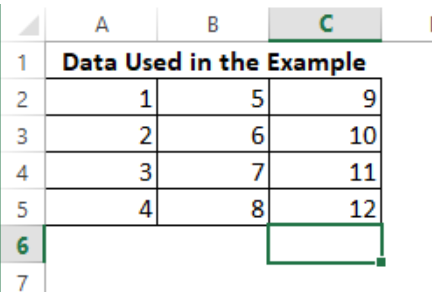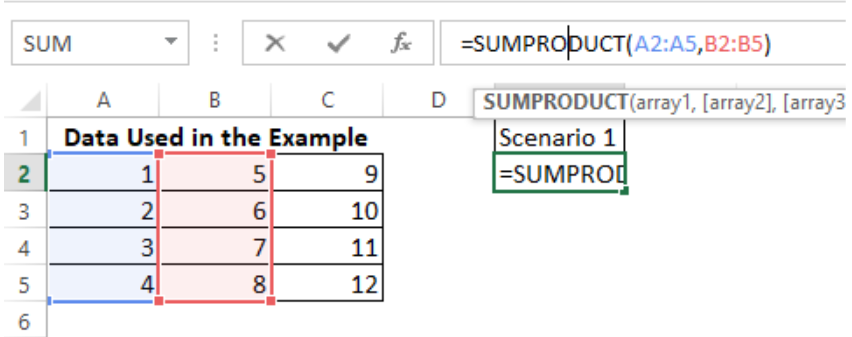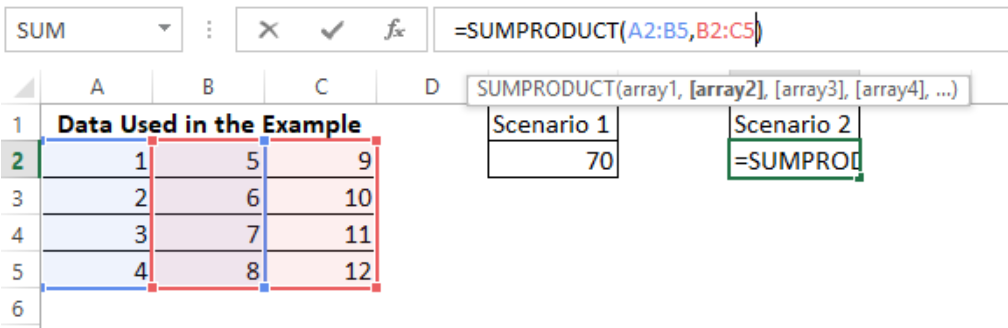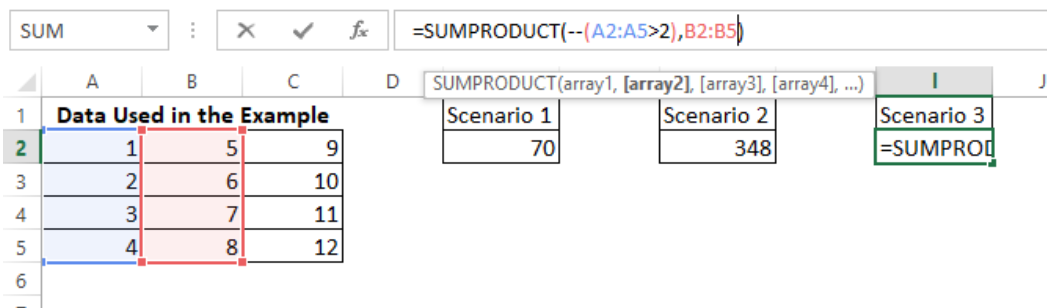Get instant live expert help with Excel or Google Sheets“My Excelchat expert helped me in less than 20 minutes, saving me what would have been 5 hours of work!”

#### Post your problem and you’ll get Expert help in seconds.

Your message must be at least 40 characters
Our professional Expert are available now. Your privacy is guaranteed.

# How to Use Excel’s SUMPRODUCT Function

If you need to multiply some corresponding components in a given array and desire to return the sum of the resulting products, then follow these quick basic steps using the SUMPRODUCT function in Excel.

## Excel SUMPRODUCT function

The formula in use here is:

`=SUMPRODUCT( array1, [array2], [array3], ... [array_n] )`

There are 2 basic steps to arrive at this result, and they are as follows:

Step 1: Prepare your Excel workbook with the array of data to be calculated

Step 2: Apply the formula as desired

### Formula for SUMPRODUCT

`=SUMPRODUCT( array1, [array2], [array3], ... [array_n] )`

Step 1:

The sample array data is created in the manner as displayed below:Figure 1- SUMPRODUCT FUNCTION

Step 2:

At this step, we will go through some series of scenarios where SUMPRODUCT function can be applied to produce results:

SCENARIO 1Figure 2 – SUMPRODUCT FUNCTION

In this first scenario, A2:A5 was used as array1 and B2:B5 was used as array2. The SUMPRODUCT function is calculated on the Cell E2, and the resulting formula is

`=SUMPRODUCT(A2:A5, B2:B5)`

The above SUMPRODUCT function calculates the selected arrays as `=(A2*B2)+(A3*B3)+(A4*B4)+(A5*B5) `and results in 70.

SCENARIO 2Figure 3- SUMPRODUCT FUNCTION

In this second scenario, A2:B5 is used as array1, and B2:C5 is used as array2. The SUMPRODUCT function is calculated on Cell G2 and the resulting formula is `=SUMPRODUCT(A2:B5, B2:C5)`

The above SUMPRODUCT function calculates the selected arrays as `=((A2*B2)+(A3*B3)+(A4*B4)+(A5*B5))+((B2*C2)+(B3*C3)+(B4*C4)+(B5*C5)) `and results in 348.

SCENARIO 3Figure 4 – SUMPRODUCT FUNCTION

In this second scenario, A2:A5 is used as array1, and B2:B5 is used as array2. The SUMPRODUCT function is calculated on Cell i2 and the resulting formula is

`=SUMPRODUCT(--(A2:A5>2),B2:B5)`

This example has a logical function where array1 checks to see data within the array that does not fulfill the logical condition before any calculation is done.

As it happens in all logical conditions, results are returned in the TRUE or FALSE format. The “–” in front of array1 is to change the logical results of TRUE or FALSE to 1 or 0 respectively. This is because the SUMPRODUCT function recognizes only numeric values.

The above SUMPRODUCT function calculates the selected arrays as explained below:

• Since A2 < 2, 0 is reallocated to A2
• Since A3 = 2, 0 is reallocated to A3
• Since A4 > 2, 1 is reallocated to A4
• Since A5 > 2, 1 is reallocated to A5.

Hence this formula is given as:

`=(A2*B2)+(A3*B3)+(A4*B4)+(A5*B5)`

Which is `= (0*5) + (0*6) + (1*7) + (1*8)`

The result is 15.

### Note

The SUMPRODUCT function can only work with numeric values, and the selection range size of array1 must be same with selection range size of array2 and on till array n.

Still need some help with Excel formatting or have other questions about Excel? Connect with a live Excel expert here for some 1 on 1 help. Your first session is always free.

Are you still looking for help with Conditional Formatting? View our comprehensive round-up of Conditional Formatting tutorials here.

### Did this post not answer your question? Get a solution from connecting with the expert.Another blog reader asked this question today on Excelchat:
Solution examplesUse the Vlookup Function to complete the "employee" column of table 2. Use "job Id" from table 2 as your lookup_value(s) and table 1 as your reference.
Solved by C. H. in 16 minsIf a cell in another sheet is populated I need a vlookup done. If the cell is not populated I need the cell to return blank.
Solved by T. D. in 60 minsI am trying to make a chart that turns a week range red if nothing is entered in the range. If something is entered then I would like it to turn green. Please Help
Solved by E. U. in 43 minsI need a check box to show/hide an answer of an if function
Solved by Z. U. in 23 minsI need a formula to compare the data in two columns and then export the mismatched data in the 3rd column
Solved by S. Q. in 20 mins## Subscribe to Excelchat.coAnother blog reader asked this question today on Excelchat: